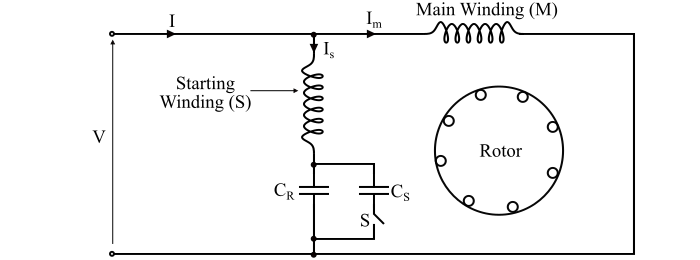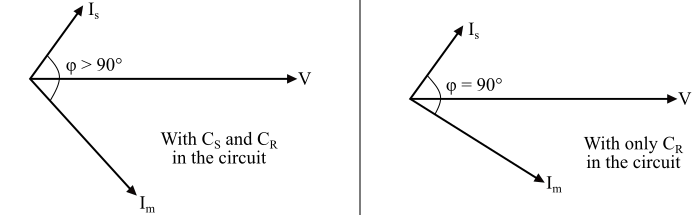# Capacitor-Start Capacitor-Run Induction Motor

The capacitor-start capacitor-run motor is a type of single-phase induction motor. The capacitor-start capacitor-run induction motor is also known as two value capacitor motor. The schematic diagram of a capacitor-start capacitorrun induction motor is shown below.The capacitor-start capacitor-run induction motor consists of a squirrel cage rotor and its stator has two windings, viz. the starting or auxiliary winding and the main or running winding. The two windings are displaced by an angle of 90° in the space.

This motor uses two capacitors − the starting capacitor (CS) and the running capacitor (CR). The two capacitors are connected in parallel at the instant of starting.

In order to obtain a high starting torque, a large starting current is required. For this, the capacitive reactance in the starting winding should be low.

Since the reactance of the starting capacitor is given by,

$$\mathrm{𝑋_{𝑆}=\frac{1}{𝜔𝐶_{𝑆}}}$$

Hence, for XS to be small, the value of starting capacitor (CS) should be large. The starting capacitor CS is a short-time rated electrolytic capacitor.

During the normal operation of the motor, the rated line current should be smaller than the starting current. Therefore, the capacitive reactance of the running capacitor should be high and is given by,

$$\mathrm{𝑋_{𝑅} =\frac{1}{𝜔𝐶_{𝑅}}}$$

Hence, for XR to be high, the value of the running capacitor (CR) should be small. The running capacitor is a long-time rated capacitor and is usually of oil-filled paper construction.

As the motor attains the normal speed, the starting capacitor (CS) is disconnected from the motor circuit by a centrifugal switch (S) and the running capacitor (CR) remains permanently connected in the circuit. Since one capacitor (CS) is used only at starting and the other capacitor (CR) for continuous running, the motor is known as capacitor-start capacitor-run motor.

The phasor diagram of the capacitor-start capacitor-run motor is shown below. At starting both the capacitors are in the circuit, therefore, the phase angle φ is greater than 90°. When the starting capacitor (CS) is disconnected from the circuit, then the phase angle becomes 90° electrical.## Characteristics of Capacitor-Start Capacitor-Run Induction Motor

Following are the primary characteristics of a capacitor-start capacitor-run induction motor −

• These motors have quiet and smooth running operation.

• They have high efficiency.

• These motors produce constant torque and not a pulsating torque.

• Because of the constant torque, the motor is vibration free.

## Applications of Capacitor-Start Capacitor-Run Motor

The capacitor-start capacitor-run motors are used for driving the loads of high inertia requiring frequent starts where the pull-out torque and the efficiency required are high such as −

• Air compressors

• Refrigerators

• Pumping equipment

• Hospitals, studios and at other places where silence is important, etc.